# Homotopy group of product is product of homotopy groups

Jump to: navigation, search

## Statement

### For two based topological spaces

Suppose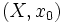$(X,x_0)$ and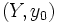$(Y,y_0)$ are based topological spaces. Then, the following is true for the homotopy groups of the topological spaces$(X,x_0)$,$(Y,y_0)$ and the product space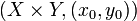$(X \times Y,(x_0,y_0))$: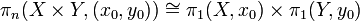$\! \pi_n(X \times Y, (x_0,y_0)) \cong \pi_1(X,x_0) \times \pi_1(Y,y_0)$

More explicitly, if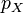$p_X$ and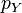$p_Y$ denote the projections from$(X \times Y,(x_0,y_0))$ to$(X,x_0)$ and$(Y,y_0)$ respectively, then the maps: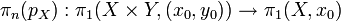$\! \pi_n(p_X): \pi_1(X \times Y,(x_0,y_0)) \to \pi_1(X,x_0)$

and: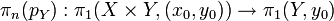$\! \pi_n(p_Y): \pi_1(X \times Y,(x_0,y_0)) \to \pi_1(Y,y_0)$

then under the isomorphism$\pi_n(X \times Y, (x_0,y_0)) \cong \pi_n(X,x_0) \times \pi_n(Y,y_0)$ we get the direct factor projections for the group product.

### For two topological spaces without basepoint specification

Suppose$X$ and$Y$ are both path-connected spaces, or more generally, each of them is a space such that all the path components of the space are homeomorphic to each other. Then, the fundamental groups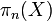$\pi_n(X)$,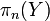$\pi_n(Y)$, and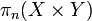$\pi_n(X \times Y)$ are all well-defined without specification of basepoint. We then have:$\! \pi_1(X \times Y) \cong \pi_1(X) \times \pi_1(Y)$